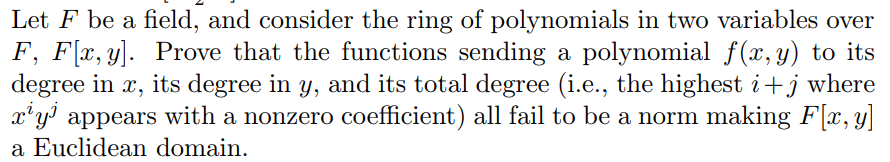e a field, and consider the ring of polynomials in two variables overF, FE, y. Prove that the functions sending a polynomial f(x, y) to itsdegree in x, its degree in y, and its total degree (i.e., the highest i +j wherea'y' appears with a nonzero coefficient) all fail to be a norm making Fl,)a Euclidean domain.

Question

Abstract Algebra. Please explain everything in detail.help_outlineImage Transcriptionclosee a field, and consider the ring of polynomials in two variables over F, FE, y. Prove that the functions sending a polynomial f(x, y) to its degree in x, its degree in y, and its total degree (i.e., the highest i +j where a'y' appears with a nonzero coefficient) all fail to be a norm making Fl,) a Euclidean domain. fullscreen
Step 1

To discuss the reason why the three given functions fail to satisfy the properties of a Euclidean norm.

Step 2

.Let D be a domain. A non-negative integer valued function N: D-{0}  is Euclidean , if given a, b in D there exist q and r in D such that a=bq+r, with either r=0 or N(r)<N(b). Basically, the function N makes the Euclidean algorithm work in D.

Step 3

To show that f(x,y) going to highest degree in x is not a Euclidean function. (By symmetry) , the...

Want to see the full answer?

See Solution

Want to see this answer and more?

Our solutions are written by experts, many with advanced degrees, and available 24/7

See Solution
Tagged in

Math